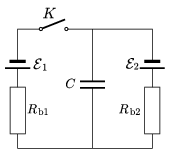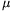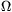Mathematical and Physical Journal
for High Schools
Issued by the MATFUND Foundation
 Already signed up? New to KöMaL?

#Problem P. 3841. (November 2005)

P. 3841. In the circuit shown in the figure switch K is turned off for a long time, then it is turned on for a long time. How does the charge of the condenser change?Data: C=10F,, Rb1=0.2,, Rb2=0.4.

(4 pont)

Deadline expired on December 12, 2005.

### Statistics:

 79 students sent a solution. 4 points: 53 students. 3 points: 8 students. 2 points: 10 students. 1 point: 4 students. Unfair, not evaluated: 4 solutionss.

Problems in Physics of KöMaL, November 2005